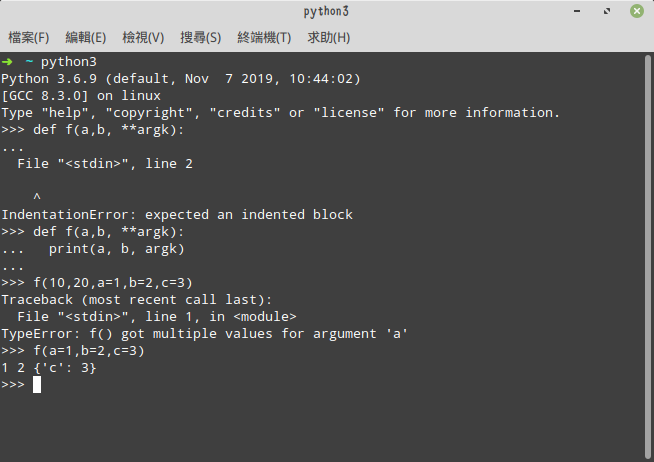# Python3.8的幾個我覺得有意思的新特性

Python 3.8同樣有不少變動，有些蠻細微的，需要詳細了解可能還是直接看Python 3.8 有什么新变化der好。現在文檔都有(簡體)中文版，覺得比英文好讀多了。所以這篇只會分享幾個我覺得有意思的新特性。

# 象牙運算符(:=)

```# @symbol var
def :=(val):
nonlocal var
var = val
return var
```

```if (n := len(a)) > 10:
print(f"List is too long ({n} elements, expected <= 10)")
```

```# Loop over fixed length blocks
while (block := f.read(256)) != '':
process(block)
```

# PEP 578: Python 運行時審核鉤子

PEP 578: Python 运行时审核钩子。這特性在開發框架比較會用到，給的訊息也不多。(但我從其他語言的觀察來看，鉤子(hook)真的很好用)

# C API 初始化配置

PEP 587: Python 初始化配置。我自己有些過一點Python提供的C API，但到時候有要再用到，再來看wwww。

# 僅位置形參

```def f(a, b, c, d, e, f):
print(a, b, c, d, e, f)
```

```f(10, 20, 30, d=40, e=50, f=60)
```

```def f(a, b, /, c, d, *, e, f):
print(a, b, c, d, e, f)
```

`a``b`就只能用位置傳遞參數，而`e``f`只能用關鍵字傳遞參數。至於`c``d`不受限制。

```def f(a, b, /, **kwargs):
print(a, b, kwargs)

f(10, 20, a=1, b=2, c=3) # a and b are used in two ways
# output: 10 20 {'a': 1, 'b': 2, 'c': 3}
```Python 3.8還有相當多細小的改動，以上就是幾個我覺得比較有意思的。

This site uses Akismet to reduce spam. Learn how your comment data is processed.